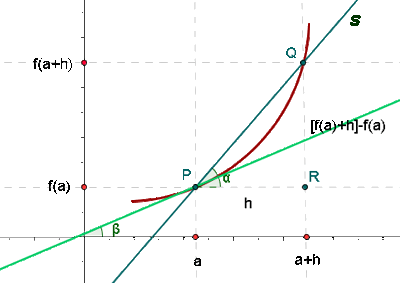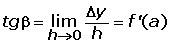## Slope of the Tangent LineThe slope of the tangent to a curve at a point is the derivative of the function at that point.The line tangent to a curve at a point is one that passes through the point (a, f(a)) and whose slope is equal to f '(a).Find the equation of the tangent to the parabola y = x² − 5x + 6 parallel to the straight line 3x + y − 2 = 0.

Point of contact: (a, f(a))

m = −3

f'(a) = 2a − 5

2a − 5 = −3a = 1

P(1, 2)

y 2 = −3 (x 1)y = −3x + 5

Did you like the article?(1 votes, average: 5.00 out of 5)Loading...

Emma

I am passionate about travelling and currently live and work in Paris. I like to spend my time reading, gardening, running, learning languages and exploring new places.

Did you like
this resource?

Bravo!